# Pointers in C Programming

C is a programming language. Like any other programming language, it uses variables in it to temporarily hold the data so that it can easily manipulate them in the code. When we say a variable, it actually takes some memory space in the system to store those values - a memory is allocated to it so that variable can store different values at each stage of the code processing. Variables are used to store input data, intermediary temporary results and final results. Variable can be of any data type and memory will be allocated accordingly. For example int will have 4 bytes of space; char will have 1 byte etc. Hence when we declare a variable as int x then C compiler allocates 4 bytes of space in memory for x to stores its integer values. This space allocated to x in turn has address which tells the compiler where exactly we can find the values of x.  It holds any integer values and it can be changed at any point while coding.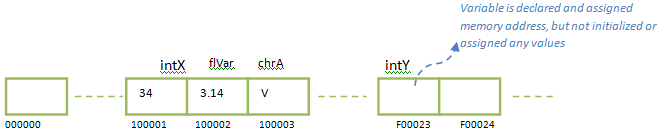Below diagram shows how a variable is stored in memory location with its data. In other words we can say a variable is a named memory location. In order to access the values stored in memory address, we call it by name rather than remembering its memory address.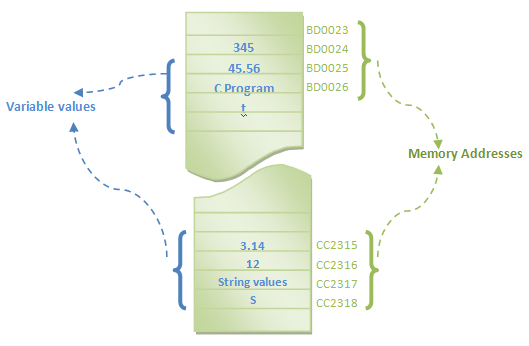Imagine what happens when a variable itself contains a memory location / address of another variable? Will it help in using it in code? The pointer is the concept that revolves around variable, its actual value and its memory address. It greatly helps in dynamic memory allocation, dynamic data structures and function variables. Let us see them in detail below.

Let us consider an example first to understand the concept of pointers. Consider we are new to a library and we want to search for ‘Let Us C’ book written by Yashavant Kanetkar.  But we do not know where it is placed in library. Hence we first search in catalogue list for its location, and then get that book for reading. Catalogue tells us where exactly each books are placed. We can see here a specific place for book in the library and a place for catalogue. Here catalogue is easily available for the visitors, and they can search for the place where the books are stored. Once visitors know the location where book is placed they can easily go there and get it. Hence catalogue is the list of place/address/shelf details of all books and the shelves are the actual location where the books are really placed. In other words catalogues are the pointers to actual location of books.
Similarly in C, pointers are the variables, which store the memory address of another variable with data. It tells the user where a variable is stored.

Below diagram shows this concept of pointers. We can see that we have variables var1, var2, var3, var4, ptr1, prt2, ptr3 and ptr4 stored in different memory addresses. Variables var1 – var4 has some string values. Variables ptr1 –ptr4 has also values but they are the addresses of var1-var4. Hence variables ptr1-ptr4 is called as pointers – points to other variables.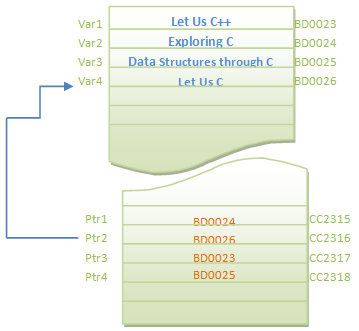To be more specific on this concept, let us discuss this in little more detail. Consider we have a variable x which is of integer type. At the beginning of the program normally we declare it.

int x;

When we declare a variable like above, a memory address is allocated to variable x to hold its value. It also indicates compiler that x can hold only integer values.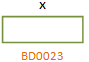Since we have only declared it, it is only assigned with memory address, but no values are stored for x. It points to NULL value currently. Let us assign value to it. Now its memory location has its value as 50. We can access this value in memory by using the variable name. Until here it is same concept as any other variable

x=50;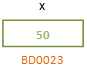Now, suppose we have another variable y, which wants to have same value as x has. Then we have to copy the value of x to y, and we can write code as y=x. But what exactly happens when we write this code? It picks the value of x from its memory location and places it in the memory location of y.

int x, y;
x=50;
y=x;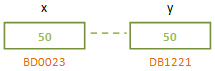Now we have same value stored at two different locations in memory. Suppose we make y as pointer to x. Then y will hold the address of x.

int x;
int *y;
y=&x;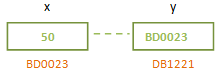Now see the difference between above two cases where y as a normal variable and as a pointer variable. How values of y differ in both cases? When it is a normal variable, it has value as any other variable stores in a memory. When y is a pointer, it also has value but it is a memory address of other variable. Thus when y used as a pointer variable, if we try to print the value of y as variable, it will print the address value stored in it. If we try to print its value as a pointer variable, then it will print the value stored at the address location that it has stored.

``````#include <stdio.h>
int main()
{
int x = 50; 	// Normal variable declaration
int *y;		 // pointer variable declaration
y = &x; 		// Address of variable x is stored in pointer variable
printf("The value of x is : %d\n", x);    // The value of x is : 50
printf("The address of x is : %d\n", &x);  // The address of x is : BD0023
printf("The address of y is : %d\n", &y);  // The address of x is : DB1221
printf("The address stored at y is : %d\n", y); // The address stored at y is : BD0023
printf("The value of *y(as a pointer) is : %d\n", *y);  // The value of *y (as a pointer) is: 50
return 0;
}
``````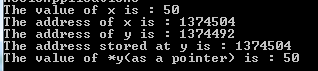Please note that the address of variable and pointer will be different each time the code is executed. In the code above, addresses are shown with respect to the diagram to have clear idea about pointers.

## Using Pointers

One may think why we have to store the address of the variable in another variable and then reference the data in it. It may look little complicated at this stage. But as we see the features of pointers in following sessions, we will really understand the power of pointers. At this point let us understand we can access the value of variable by using that variable itself or by using a pointer variable that it is pointed by. In our example above we can access 50 by using variable x or by using pointer variable *y.

Whenever a variable is a normal variable, then it is denoted by its name. But when a variable is declared as a pointer variable, ‘*’ is appended to the variable name at the beginning. This denotes the compiler that it is not a normal variable, but it is a pointer variable. E.g.; x is a normal variable and *x is a pointer variable.

## Declaring Pointers

Like any other variable, pointer is also a variable. Hence we need to declare it before using it in the code. It is also declared as a normal variable. But * appended to it tells the compiler that it is a pointer variable. This is the only difference between declaring normal variable and pointer variable.

``````datatype *variable_name;  //general declaration of pointer

int intX;
int *intPtr;
float *flPtr;
char *chrPtr;
``````

In above examples we can see pointers are declared as integer, float, and character. One may think how a pointer can be of different datatypes, when it is used to store address of another variable. But pointer variables works little different here. When we say integer pointer, it means the pointer is pointing to integer variables and when it is used along with ‘*’, it shows the integer values of the variable that it is pointing to. Similarly, when we say float pointer, then it is pointing to float variables and displays float values. So is the case with character pointer. This concept is clear from below diagram. We can see that intX is a variable which holds integer value and intPtr is pointing to address of intX. Hence when we say: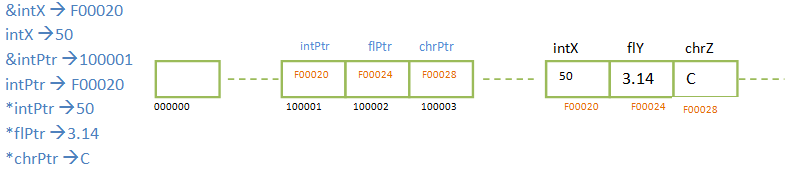Hence by default any pointer variable will hold memory address and we need not specify datatype for it. But it is very much necessary to specify the datatype that a pointer is pointing to. In addition, it also tells the compiler that when*intPtr is used, it is pointing to integer and it should allocate 4 bytes of memory to it. When *chrPtr is used, compiler understands 1 byte of memory is enough to store the data. When pointer arithmetic like increment or decrement is used it actually increases / decreases the memory addresses. Hence such cases it increases / decreases the number of memory blocks according to the datatypes used. For example, suppose a character pointer is incremented by 1. Then the compiler knows that for a character pointer only one byte of memory is needed and increases the address by 1 byte. But when an integer pointer is incremented(say, initially it pointing to F00020), compiler increments the memory address by 4 (now it will point to F00024)as each integer occupies 4 bytes of space. Hence we need to specify the datatype for pointer which will help in determining the memory blocks for the pointers.

Suppose we have declared variables as shown below :

int   *intPtr, X;

What does above declaration mean – both the variables as pointer? No. Only *intPtr is a pointer variable, whereas X is a normal variable. Does below declaration declare both variables as pointers ?

int*   intPtr, X;

No. The declaration syntax above looks like it is declaring both the variables as pointers. But it is same as first declaration – where intPtr is a pointer and X is a normal variable. If we need to declare both of them as pointer, then we need to specify ‘*’ before each variable as shown below:

int *intPtr, *X; // now both the variables are pointers

Another option for declaring more than one pointer variable is to declare them in separate lines.

``````int *intPtr;
int *X;``````

Pointers need not be pointing to normal variables like integer, character, float etc. It can also point to arrays, structures, functions too. Pointers pointing to other complex datatypes are declared in the same way we declared pointers above with ‘*’.

``````int   *intArrPtr ; // it is an array of pointers with 10 elements of integer type
float   *fltArrPtr ; // it is an array of pointers with 10 elements of float type
``````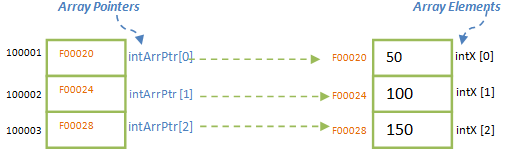We can see above that array elements occupy consecutive memory address and have same datatypes. The pointer is intArrPtr is array of pointers, which holds the address of each elements of the array as shown in above diagram.  Each element of the array can be referenced in the same way as array as shown below. This type of declaring the array pointer is known as array of pointers. Let us discuss more details of array pointers in ‘Array Pointers’ section below.

``````intArrPtr = F00023        *intArrPtr = 50
intArrPtr = F00023        *intArrPtr = 100
intArrPtr = F00023        *intArrPtr = 150``````

Structure pointers are declared as shown below. Here newStruct is a structure with variables of same/ different datatype. When we declare a pointer to structure it points to the memory address of the structure (usually beginning of the structure). More details about these type pointers are discussed at ‘Pointers to Structure’ section below.

struct newStruct *ptrStruct; //structure pointer to a newStruct structure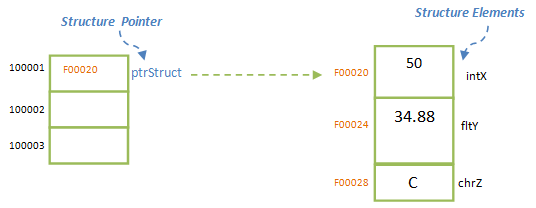We can have pointers to functions too. We can declare a function pointer as shown below:

``````void (*fnPtr) (int);
void (*fnPtr) (int, char);
int (*fnPtr) (int, char);``````

We can even have pointers to pointers. It can be declared as below.

``````datatype **pointer_variable; // double star is added to indicate pointer to pointer
int **intPtrToPtr; ``````

This is how we declare different types of pointers. More details on using them are discussed in below sections.

Scroll to Top